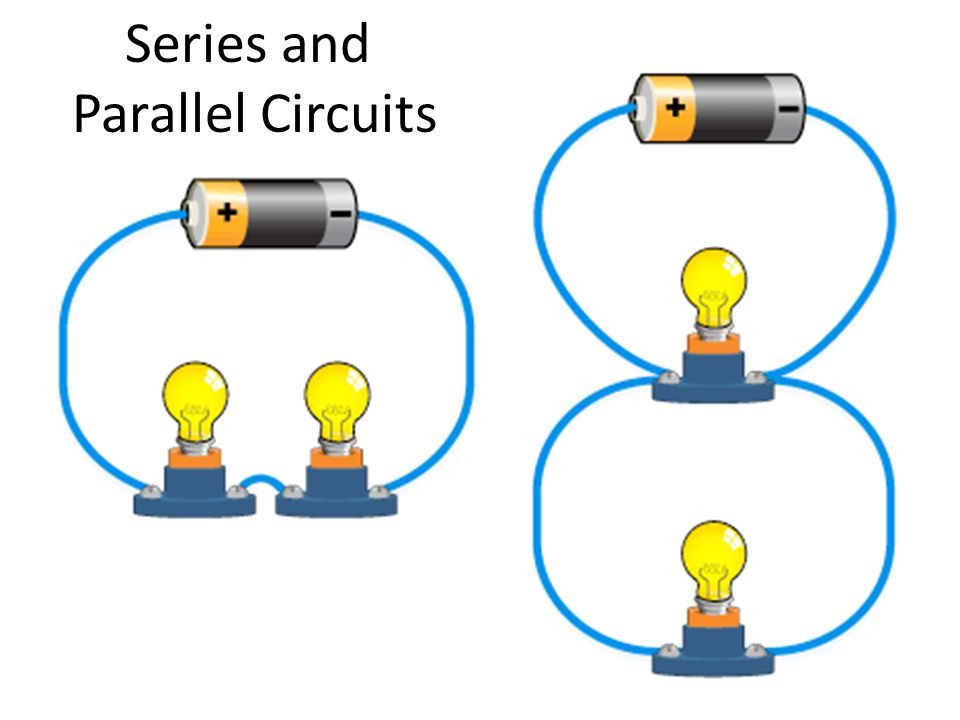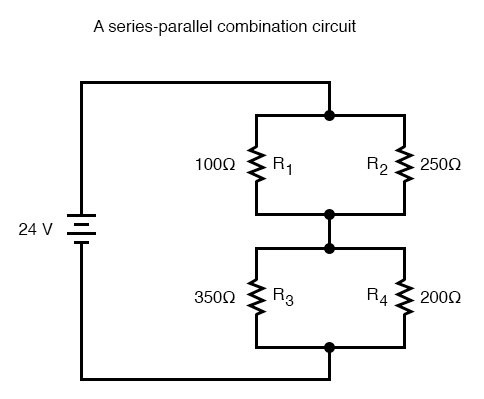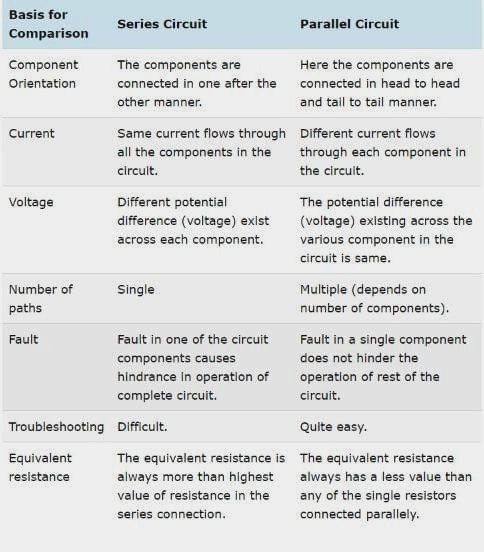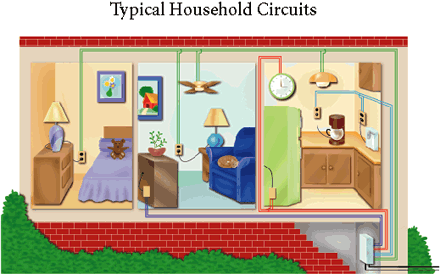• Poornima Mani

# Series and Parallel Circuits

Updated: Dec 1, 2020

In this article, we will look into the most commonly used and easy to understand the types of circuits in #electronics: Series and Parallel Circuits.### Series Circuits

Series #circuits involve all the resistors, loads, or components connected end-to-end thus enabling only one path for the #current to flow through. Let us understand this with the help of a circuit.Series Circuit

In this image, we can see multiple resistors named R1, R2, and R3 connected as a chain from the source. This shows that there is only one path for current to flow from one point to another in the entire circuit.

The resistance value in a series circuit can be identified by simply adding all of it together.

In this case, let us assume that the values are R1=8 Ohm, R2=8 Ohm, and R3=4 Ohm. The total resistance will be 20 Ohm.

### Parallel Circuits

A parallel circuit involves more than one component connected by parallel wires.Parallel Circuit

The specialty in a parallel circuit is that the voltage is sane across the circuit, though the current in each branch may vary.

In such a circuit, we find the resistance by adding the reciprocal of the resistances or using the equation :

1 / R = 1 / R1 + 1 / R2 + 1 / R3

in this case.

Considering the same resistances as the previous case, i.e. 8 Ohm, 8 Ohm and 4 Ohm for R1, R2 and R3 respectively, the resistance is obtained as 2 Ohm.

But Series and Parallel combinations are not confined to this. There can be diverse possibilities which include series and parallel connections in a single circuit. Let us check out this concept first.

### Series-Parallel Configuration

This is the most practical configuration as it is not technically possible to use series and parallel circuits alone always. But understanding both the concept basics is important to thoroughly know the Series-Parallel configuration.In this circuit, we can see that resistors R1 and R2 are connected in parallel, and R3 and R4 are also connected in parallel. But these are connected in series with each other.

So, in such cases, the order can be decided and the value could be found out accordingly.

So how are you will you find the values?

First, calculate the resistance in R1 and R2 and then the resistance in R3 and R4. After that, calculate the series resistance between these. Easy right?

But let us just take a look at a realistic circuit that will blow your mind.Breadboard with series and parallel connections

### Comparison between series and parallel circuits### Applications

Series circuits are low power circuits and voltage divider circuits. But parallel circuits are the ones that are most commonly used.

Can you guess what kind of circuit will be used in our households?

YES! Parallel Circuits. This is because, in households, there will be multiple appliances connected to a single network and if there happens to be any kind of power fluctuation in any of the devices, that could potentially create issues in the entire household and may even lead to an unstoppable disaster. Hence, every home has parallel networks.Series:

• Series circuits are easy to design and be built.

• Fuse is connected in series to stop the current and prevent the excess flow of electricity through it.

• It is less expensive compared to a parallel circuit.Fuse connected in series to the power supply

Parallel:

• The major advantage is that no matter how many numbers of devices or appliances are added, there isn't any issue with the circuit.

• In this kind of circuit, the voltage remains the same throughout.

• It is most commonly used in almost all practical applications.

Series:

• If at least one of the load is damaged, then the entire circuit becomes irresponsive.

• As the number of load increases, the voltage divided in them is reduced. For eg., if there are multiple light bulbs connected in series, then you could see that the gets dimmer.

• It is not suitable for practical applications.

Parallel:

• It is more complicated to design a parallel circuit.

• It is more costly.

• The chances of a short circuit in any of the branch is much higher and could be hazardous.